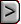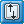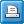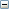﻿ PCGN - Preconditioned Conjugate Gradient Solver with Improved Nonlinear Control
Online Guide to MODFLOW

PCGN - Preconditioned Conjugate Gradient Solver with Improved Nonlinear ControlPCGN - Preconditioned Conjugate Gradient Solver with Improved Nonlinear ControlPCGN - Preconditioned Conjugate Gradient Solver with Improved Nonlinear ControlAbbreviation in Name file

PCGN

Purpose

The Preconditioned Conjugate Gradient Solver with Improved Nonlinear Control package is used to solve the finite difference equations in each step of a MODFLOW stress period.

Documentation

Naff, R.L., and Banta, E.R., 2008, The U.S. Geological Survey modular ground-water model—PCGN: A preconditioned conjugate gradient solver with improved nonlinear control: U.S. Geological Survey Open-File Report 2008–1331, 35 p.

https://wwwbrr.cr.usgs.gov/projects/GW_stoch/pcgn/index.shtml

Related Packages

Solvers

Supported in

 • MODFLOW-2000
 • MODFLOW-2005
 • MODFLOW-LGR
 • MODFLOW-OWHM

Other Notes

 • As of Mar. 28, 2012, the PCGN solver was not included in the versions of MODFLOW-2000 and MODFLOW-LGR released via https://water.usgs.gov/software/.  Versions of MODFLOW-2000  and MODFLOW-LGR that contains the PCGN package can be downloaded from https://wwwbrr.cr.usgs.gov/projects/GW_stoch/pcgn/index.shtml.
 • If the Lake package is used, more iterations may be required.

The PCGN solver package is invoked by inserting the file type “PCGN” in the MODFLOW Name file (Harbaugh and others, 2000). This entry in the Name file also is used to associate the file type “PCGN” with the name of a file from which the input values for the PCGN solver package are read; these input values are described in this section. Either fixed or free format is available for reading all values on the input list. The PCGN data file should contain the following data items in the order given:

1.        ITER_MO, ITER_MI, CLOSE_R, CLOSE_H

2.        RELAX, IFILL, UNIT_PC, UNIT_TS

3.        ADAMP, DAMP, DAMP_LB, RATE_D, CHGLIMIT

4.        ACNVG, CNVG_LB, MCNVG, RATE_C, IPUNIT

Optional comments may be added to the input data file by preceding the comment with the symbol # in the first column; these comments may appear anywhere in the data file. If fixed format is selected, then the corresponding format types, for the preceding variables, are as follows:

1.        2I10,2F10.0

2.        F10.0,3I10

3.        I10,4F10.0

4.        I10,F10.0,I10,F10.0,I10

The variables on the line 1 above generally manage the inner and outer iterations and overall convergence of the problem. The line 2 variables generally pertain to the PCG solver. The line 3 variables generally manage the damping applied when updating a nonlinear problem, and the line 4 variables manage the convergence of the PCG solver.

Generally speaking, if little is known of the characteristics of the nonlinear problem, one is advised to start the modeling process with ACNVG = 0, ADAMP = 0 and DAMP = 0.5; if damping at this level does not produce convergence of the nonlinear problem, then damping should be reduced, in the extreme to the DAMP = 0.01 level. If convergence is still not achieved, and the Picard iteration progress report (IPUNIT > 0) indicates that residuals are not being reduced, then the modeler should use enhanced convergence (ACNVG = 2, MCNVG > 1) with no variation allowed (RATE_C ≤ 0). If difficulties persist, then the modeler may wish to attempt adaptive damping (ADAMP = 1) with small starting and limiting parameters: DAMP_LB = 0.001 and DAMP = 0.1. Memory usage permitting, convergence of the Picard iteration might also be aided if fill level IFILL = 1 can be used. If any of these modes produce a Picard solution with a reasonable mass balance, then the modeler will likely wish to refine these modes so as to increase the efficiency and accuracy of the Picard iteration.

Results thus far of testing the PCGN package on various nonlinear problems have not indicated that any particular set of Picard parameters have preference. Some nonlinear problems simply will not converge without use of adaptive damping, although simultaneous use of the standard convergent scheme is frequently adequate. Convergence of dewatering problems is frequently enhanced if a bound for the maximum head change, CHGLIMIT, can also be instituted. On the other hand, several nonlinear problems showed a strong preference to increasing the accuracy of the linear solution by allowing ACNVG > 0. Here, the ACNVG = 1 option was generally found to be less useful than the ACNVG = 2 option when used in conjunction with RATE_C set to a small positive value. That is, using an option that initially tightens the relative convergence criterion ε, but slowly relaxes it so that standard convergence is gradually reestablished, is more likely to enhance performance of the solver. In dewatering simulations, the ACNVG = 2 option frequently diminishes the number of cells that go dry. That is, having a slightly more rigorous solution to the linear approximation early in the Picard iteration may decrease the number of cells that go dry.

With regard to the Picard parameters (ITER_MO > 1), the PCGN package does limited checking to ascertain that the input data are consistent and correct. If an inconsistent or out of range entry is encountered, the package usually resets ADAMP and (or) ACNVG to 0, issues a warning, and allows the computation to continue. The user must examine the MODFLOW Listing file to detect such warnings; if such warnings are found, the PCGN data input file should be examined for errors.

FOR EACH SIMULATION

Data Set 1

ITER_MO, ITER_MI, CLOSE_R, CLOSE_HExplanation of variablesITER_MO
 ITER_MO, integer variable: ITER_MO is the maximum number of Picard (outer) iterations allowed. For nonlinear problems, this variable must be set to some number greater than one, depending on the problem size and degree of nonlinearity. If ITER_MO is set to 1, then the PCGN solver assumes that the problem is linear and the input requirements are greatly truncated.ITER_MI
 ITER_MI, integer variable: ITER_MI is the maximum number of PCG (inner) iterations allowed. Generally, this variable is set to some number greater than one, depending on the matrix size, degree of convergence called for, and the nature of the problem. For a nonlinear problem, ITER_MI should be set large enough that the PCG iteration converges freely with the relative convergence parameter ε described in the Parameters Related to Convergence of Inner Iteration: Line 4 subsection.CLOSE_R

CLOSE_R, real variable: CLOSE_R is the residual-based stopping criterion for iteration. This parameter is used differently, depending on whether it is applied to a linear or nonlinear problem:

 • ITER_MO = 1: For a linear problem, the variant of the conjugate gradient method outlined in algorithm 2 is employed, but uses the absolute convergence criterion in place of the relative convergence criterion. CLOSE_R is used as the value in the absolute convergence criterion for quitting the PCG 9 iterative solver. CLOSE_R is compared to the square root of the weighted residual norm ν. This norm is defined as ν = rTM−1r , where M represents the preconditioning matrix with the PCG algorithm and r is a vector of residuals. In particular, if √ν is less than CLOSE_R, then the linear PCG iterative solve is said to have converged, causing the PCG iteration to cease and control of the program to pass out of the PCG solver.
 • ITER_MO > 1: For a nonlinear problem, CLOSE_R is used as a criterion for quitting the Picard (outer) iteration. CLOSE_R is compared to the square root of the inner product of the residuals (the residual norm), [rT r]1/2 , as calculated on entry to the PCG solver at the beginning of every Picard iteration. If this norm is less than CLOSE_R, then the Picard iteration is considered to have converged.CLOSE_H
 CLOSE_H, real variable: CLOSE_H is used as an alternate stopping criterion for the Picard iteration needed to solve a nonlinear problem. The maximum value of the head change is obtained for each Picard iteration, after completion of the inner, PCG iteration. If this maximum head change is less than CLOSE_H, then the Picard iteration is considered tentatively to have converged. However, as nonlinear problems can demonstrate oscillation in the head solution, the Picard iteration is not declared to have converged unless the maximum head change is less than CLOSE_H for three Picard iterations. If these Picard iterations are sequential, then a good solution is assumed to have been obtained. If the Picard iterations are not sequential, then a warning is issued advising that the convergence is conditional and the user is urged to examine the mass balance of the solution.

As convergence of the Picard iteration may be acheived by meeting either the CLOSE_R or the CLOSE_H criterion, care must be taken in their selection. One should, in any case, check the mass balance of the solution for the problem in question to verify that a reasonable result has been obtained. Maximum head-change values at convergence are generally two to four order of magnitude smaller than the residual norm [rT r]1/2

Data Set 2

RELAX, IFILL, UNIT_PC, UNIT_TSExplanation of variablesRELAX
 RELAX, real variable: RELAX is the so-called relaxation parameter for the modified incomplete Cholesky (MIC) preconditioner (see algorithms 7 and 11); under MIC preconditioning, row sum agreement between the original matrix and the preconditioning matrix is created by pivot modification. When RELAX = 0, then the MIC corresponds to the ordinary incomplete Cholesky preconditioner, the effect of the modifications to the incomplete Cholesky having been nullified. When RELAX = 1, then these modifications are in full force. Generally speaking, it is of advantage to use the modifications to the incomplete Cholesky algorithm; a value of RELAX such that 0.9 < RELAX < 1 is generally advised for most problems. Values RELAX = 1 are not advised, particularly when IFILL = 0, as poor performance of the PCG solver may result (van der Vorst, 2003). However, experience has shown that a value close to 1, such as RELAX = 0.99, usually provides good performance.IFILL
 IFILL, integer variable: IFILL is the fill level of the MIC preconditioner. Preconditioners with fill levels of 0 and 1 are available (IFILL = 0 and IFILL = 1, respectively). Generally, the higher the fill level, the more preconditioning imparted by a MIC preconditioner. However, the actual preconditioning provided is also influenced by the modification to the incomplete Cholesky algorithm (see RELAX above). For most well-behaved CCFD matrices, a MIC preconditioner with fill level 0 will slightly outperform a MIC preconditioner with fill level 1, provided RELAX ≈ 1. For problems where the matrix equation is not well behaved or for a nonlinear problem where convergence is not easily achieved, a fill level of 1 may provide the additional preconditioning necessary to obtain convergence. One should be aware that the PCGN solver computer memory requirements of the level 1 MIC preconditioner are about double those of the level 0 preconditioner.UNIT_PC
 UNIT_PC, integer variable: UNIT_PC is the unit number of an optional output file where progress for the inner PCG iteration can be written. Progress diagnostics consist of the weighted residual norm νi for every iteration i of the PCG solver; this information is ouput for every time step and every Picard iteration in the simulation. If this option is used (UNIT_PC > 0), the integer value of the unit, along with the file name and type “DATA,” should be given in the MODFLOW Name file (Harbaugh and others, 2000). In many instances, asking for this information will cause very large data files to be produced; it is not expected that this option will be used by most modelers.UNIT_TS
 UNIT_TS, integer variable: UNIT_TS is the unit number of an optional output file where the actual time in the PCG solver is accumulated. The object here is to capture actual PCG solver time rather than total run time. If this option is used (UNIT_TS > 0), the integer value of the unit, along with the file name and type “DATA,” should be given in the MODFLOW Name file. It is not expected that this option will be used by most modelers.

If ITER_MO = 1, then no further data are read (or needed) by the PCGN package to solve a linear problem. If the problem is nonlinear (ITER_MO > 1), then the remaining two lines (3 and 4) of data are read and processed.

Data Set 3Explanation of variablesADAMP

ADAMP, integer variable: ADAMP defines the mode of damping applied to the linear solution. In general, damping determines how much of the head changes vector Δj shall be applied to the hydraulic head vector hj in Picard iteration j: hj = hj−1 +θΔj, where θ is the damping parameter. The available damping modes are:

 • ADAMP = 0: Ordinary damping is employed and a constant value of damping parameter θ = DAMP will be used throughout the Picard iteration. This option requires a valid value for DAMP.
 • ADAMP = 1: Adaptive damping is employed; see algorithm 1. Adaptive damping changes the damping parameter θ in response to the difficulty the nonlinear solver encounters in solving a given problem. Essentially, the nonlinear solver looks to increase θ should the convergence of the Picard iteration proceed satisfactorily, but otherwise causes θ to decrease. Adaptive damping can be useful for problems that do not converge readily, but otherwise should be avoided as it generally requires more total iterations. This option requires valid values for variables DAMP, DAMP_LB, RATE_D, and CHGLIMIT. Adaptive damping also admits the possibility of directly limiting the the maximum head change applicable to update the hydraulic heads; see CHGLIMIT below. If this option is not desired, then CHGLIMIT should be set to zero.
 • ADAMP = 2: Enhanced damping algorithm in which the value of θ is increased (but never decreased) provided the Picard iteration is proceeding satisfactorily. This enhanced damping allows θ to increase from a minimum value to a maximum value DAMP by a rate equal to RATE_D. The minimum value in the first stress period is DAMP_LB; for subsequent stress periods it is the geometric mean of DAMP and DAMP_LB. This option requires valid values for DAMP, DAMP_LB, and RATE_D.DAMP

DAMP, real variable: The varible DAMP restricts the damping parameter θ; generally, 0 < DAMP < 1. Its function for the various modes of ADAMP are:

 • ADAMP = 0: The damping parameter θ takes on the value DAMP and is maintained constant throughout the simulation.
 • ADAMP > 0: The value of DAMP will be treated as the upper limit for θ in the enhanced damping or adaptive damping algorithms.DAMP_LB

DAMP_LB, real variable: DAMP_LB represents a bound placed on θ; generally, 0 < DAMP_LB < DAMP. For the various modes of ADAMP > 0, DAMP_LB serves the following purposes:

 • ADAMP = 1: In the adaptive damping algorithm, DAMP_LB represents the lower limit to which θ, under adverse adaptive damping conditions, will be allowed to fall.
 • ADAMP = 2: In the enhanced damping algorithm, DAMP_LB is the starting value (or a component of the starting value) for the damping parameter θ used in the initial Picard iteration of every stress period.RATE_D

RATE_D, real variable: This variable is a rate parameter; generally, 0 < RATE_D < 1. For the various modes of ADAMP > 0, RATE_D serves the following purposes:

 • ADAMP = 1: RATE_D sets the recovery rate for the damping factor θ in response to the progress in the Picard iteration; it also forms a limit on the response function to progress in the Picard iteration. See algorithm 1 for usage when ADAMP = 1; in this algorithm, RATE_D = ψ. Typical values for RATE_D, under this scenario, are 0.01 < RATE_D < 0.1. Under adaptive damping, if the user finds that the damping factor θ increases too rapidly, then reducing RATE_D will slow the rate of increase.
 • ADAMP = 2: Provided the Picard iteration is progressing satisfactorily, RATE_D adjusts the damping factor θ upward such that θj = θj−1+ RATE_D θj−1, where j is the Picard iteration number. Typical values for RATE_D, under this scenario, are 0.01 < RATE_D < 0.1, although larger or smaller values may be used.CHGLIMIT
 CHGLIMIT, real variable: This variable limits the maximum head change applicable to the updated hydraulic heads in a Picard iteration. Provided that the current damping factor is greater than the ratio of CHGLIMIT to the maximum head change and that this ratio is less than one, then the damping factor is reset to the value of the ratio. This option is available only in association with adaptive damping: ACNVG = 1. If CHGLIMIT = 0.0, then adaptive damping proceeds without this feature.

Data Set 4

ACNVG, CNVG_LB, MCNVG, RATE_C, IPUNITExplanation of variablesACNVG

ACNVG, integer variable: ACNVG defines the mode of convergence applied to the PCG solver. In general, the relative stopping criterion for PCG iteration is νi < εν0, where ν0 is the weighted residual norm on entry to the PCG solver, ε is the relative convergence parameter, and νi is the same norm at PCG iteration i. The available convergence modes are:

 • ACNVG = 0: The standard convergence scheme is employed using the variant of the conjugate gradient method outlined in algorithm 3. The standard relative convergence is denoted by εs and usually takes the value 0.1; this value is assigned to the relative convergence ε. No additional variables are used.
 • ACNVG = 1: Adaptive convergence is employed using the variant of the conjugate gradient method outlined in algorithm 2. The adaptive convergence scheme adjusts the relative convergence ε of the PCG iteration based on a measure of the nonlinearity of the problem. Under this scheme, ε is allowed to vary such that CNVG_LB < ε < εs, where the exact value of ε is dependent on the measure of nonlinearity. This option requires a valid value for variable CNVG_LB.
 • ACNVG = 2: Enhanced convergence is employed using variant of the conjugate gradient method outlined in algorithm 3. If the variable enhancement option is employed (RATE_C > 0), then εs is taken as the upper limit for ε; see Limiting the Inner Iteration subsection for details. This option requires valid values for variables MCNVG and RATE_C.CNVG_LB
 CNVG_LB, real variable: Variable CNVG_LB is used only in convergence mode ACNVG = 1. CNVG_LB is the minimum value that the relative convergence ε is allowed to take under the self-adjusting convergence option. The objective here is to prevent ε from becoming so small that the PCG solver takes an excessive number of iterations. Valid range for variable: 0 < CNVG_LB < εs; a value of CNVG_LB = 0.001 usually produces reasonable results.MCNVG
 MCNVG, integer variable: Variable MCNVG is used only in convergence mode ACNVG = 2. MCNVG increases the relative PCG convergence criteria by a power equal to MCNVG; that is, letting p = MCNVG, then the relative convergence criterion ε is enhanced such that ε = εsp, where 0 < p ≤ 6. If MCNVG is set to a value greater than 6, then PCGN resets MCNVG = 6 and issues a warning message. If RATE_C = 0, then this enhanced relative convergence criterion is applied uniformly throughout the simulation; the relative convergence, in this case, is not adjusted for stress changes in the simulation. MCNVG must be set to a value greater than zero when ACNVG = 2; otherwise a data error is declared and ACNVG is reset to zero.RATE_C
 RATE_C, real variable: Variable RATE_C is used only in convergence mode ACNVG = 2; this option results in variable enhancement of ε. If 0 < RATE_C < 1, then enhanced relative convergence is allowed to decrease by increasing ε as follows: εj = εj−1+ RATE_C εj−1, where j is the Picard iteration number; this change in ε occurs so long as the Picard iteration is progressing satisfactorily. If RATE_C ≤ 0, then the value of ε set by MCNVG remains unchanged through the Picard iteration. It should be emphasized that RATE_C must have a value greater than 0 for the variable enhancement to be effected; otherwise ε remains constant. The assumption here is that a better solution of the linear equations is needed initially to improve the nonlinear Picard solution, but that this need abates with additional Picard iterations. Typical values for RATE_C are 0.01 < RATE_C < 0.1, although larger or smaller values may be used.IPUNIT
 IPUNIT, integer variable: Variable IPUNIT enables progress reporting for the Picard iteration. If IPUNIT ≥ 0, then a record of progress made by the Picard iteration for each time step is printed in the MODFLOW Listing file (Harbaugh and others, 2000). This record consists of the total number of dry cells at the end of each time step as well as the total number of PCG iterations necessary to obtain convergence. In addition, if IPUNIT > 0, then extensive diagnostics for each Picard iteration is also written in comma-separated format to a file whose unit number corresponds to IPUNIT; the name for this file, along with its unit number and type “DATA,” should be entered in the MODFLOW Name file. Diagnostics output by this last option are given in the Output Diagnostics for the Picard Iteration section of this report. If IPUNIT < 0 then printing of all progress concerning the Picard iteration is suppressed, as well as information on the nature of the convergence of the Picard iteration.